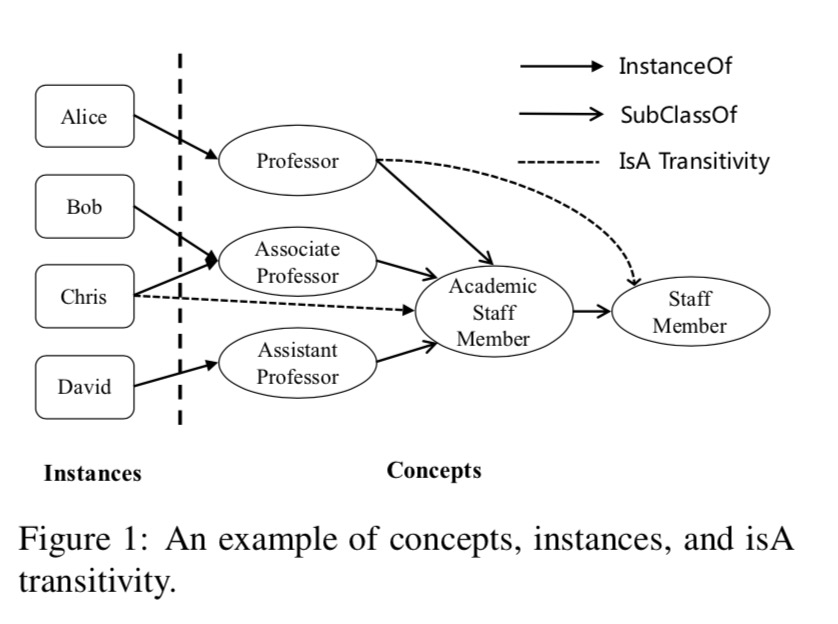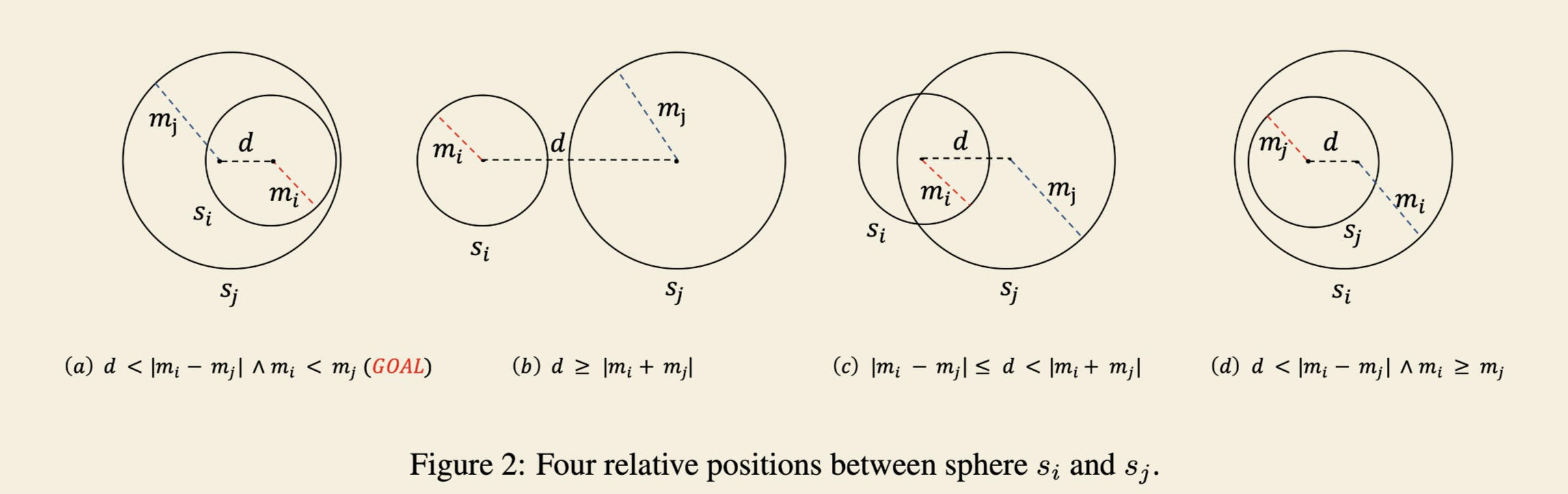《Differentiating Concepts and Instances for Knowledge Graph Embedding》阅读笔记

### concept

A concept is a fundamental category of existence (Rosch, 1973) and can be reified by all of its actual or potential instances.Concepts, which represent a group of different instances sharing common properties, are essential information in knowledge representation.## drawback of the previous method

ignore to distinguish between concepts and instances will lead to two drawbacks:

• Insufficient concept representation

cannot explicitly represent the difference between concepts and instances

• Lack transitivity of both isA relations:

instanceOf and subClassOf (generally known as isA)isA relations exhibit transitivity

## contributions

• the first to propose and formalize the problem of knowledge graph embedding which differentiates between concepts and instances
• a novel knowledge embedding method named TransC
• state-of-the-art on link prediction and triple classification

## Translation-based Models

### TransE

• triple (h, r, t) should satisfy h + r ≈ t
• loss function:$f_r(h,t) = ||h + r - t||^2_2$
• suitable for 1-to-1 relations

### TransH

• It regards a relation vector r as a translation on a hyperplane with $w_r$ as the normal vector.
• loss function:$f_r(h,t) = ||h_{\bot} + r - t_{\bot}||^2_2$，其中$h_{\bot}=h-w^{\top}_r h w_r$，$t_{\bot}=t-w^{\top}_r t w_r$
• suitable for 1-to-N, N-to-1, and N-to-N relations

### TransR/CTransR

• addresses the issue in TransE and TransH that some entities are similar in the entity space but comparably different in other specific aspects.
• loss function:$f_r(h,t) = ||M_rh +r -M_rt||^2_2$，$M_r$ for each relation r

### TransD

• considers the different types of entities and relations at the same time
• loss function:$f_r(h,t) = ||h_{\bot} + r - t_{\bot}||^2_2$，$h_{\bot} = M_{rh}h$和$t_{\bot} = M_{rt}t$，$M_{r,e}$ for each relation-entity pair (r, e)

## Bilinear Models

### RESCAL

• the first bilinear model
• It associates each entity with a vector to capture its latent semantics. Each relation is represented as a matrix which models pairwise interactions between latent factors.

## External Information Learning Models

• textual information
• entity descriptions

## Problem Formulation

For each concept c ∈ C, we learn a sphere s(p, m) with $p \in R^k$ and m denoting the sphere center and radius.

## TranC

Specifically, TransC encodes each concept in knowledge graph as a sphere and each instance as a vector in the same semantic space.

### InstanceOf Triple Representation

loss function：$f_e(i,c) = ||i-p||_2 - m$，当该函数大于0时进行优化，使其小于零。

### SubClassOf Triple Representation## train model

### unit and bern

Regarding the strategy of constructing negative labels, we use “unif” to denote the traditional way of replacing head or tail with equal probability, and use “bern.” to denote reducing false negative labels by replacing head or tail with different probabilities.

## the following research directions

• find a more expressive model instead of spheres to represent concepts

• A concept may have different meanings in different triples.

use several typical vectors of instances as a concept’s centers to represent different meanings of a concept.

上一篇《Learning Phrase Representations using RNN Encoder–Decoder for Statistical Machine Translation》阅读笔记

2019-01-05A Discourse-Level Named Entity Recognition and Relation Extraction Dataset for Chinese Literature Text 阅读笔记

2018-12-10
目录